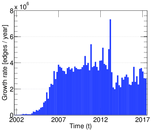# Wikipedia edits (pl)

This is the bipartite edit network of the Polish Wikipedia. It contains users and pages from the Polish Wikipedia, connected by edit events. Each edge represents an edit. The dataset includes the timestamp of each edit.

 Code `pl` Internal name `edit-plwiki` Name Wikipedia edits (pl) Data source http://dumps.wikimedia.org/ AvailabilityDataset is available for download Consistency checkDataset passed all tests Category Authorship network Dataset timestamp 2017-10-20 Node meaning User, article Edge meaning Edit Network formatBipartite, undirected Edge typeUnweighted, multiple edges Temporal dataEdges are annotated with timestamps

## Statistics

 Size n = 2,872,213 Left size n1 = 207,781 Right size n2 = 2,664,432 Volume m = 40,744,770 Unique edge count m̿ = 21,219,204 Wedge count s = 1,513,152,144,859 Claw count z = 280,394,629,893,772,576 Maximum degree dmax = 1,835,021 Maximum left degree d1max = 1,835,021 Maximum right degree d2max = 106,429 Average degree d = 28.371 7 Average left degree d1 = 196.095 Average right degree d2 = 15.292 1 Average edge multiplicity m̃ = 1.920 18 Size of LCC N = 2,818,305 Diameter δ = 13 50-Percentile effective diameter δ0.5 = 3.347 54 90-Percentile effective diameter δ0.9 = 3.925 93 Median distance δM = 4 Mean distance δm = 3.593 75 Gini coefficient G = 0.847 442 Balanced inequality ratio P = 0.163 251 Left balanced inequality ratio P1 = 0.039 452 1 Right balanced inequality ratio P2 = 0.225 381 Relative edge distribution entropy Her = 0.748 081 Degree assortativity ρ = −0.081 780 1 Degree assortativity p-value pρ = 0.000 00 Spectral separation |λ1[A] / λ2[A]| = 1.231 14

## Plots

### Degree distribution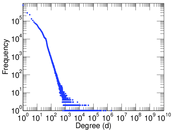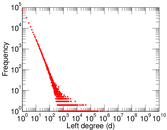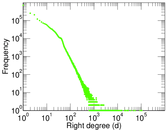### Cumulative degree distribution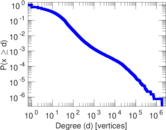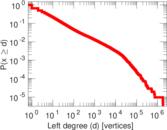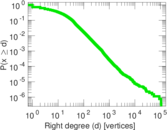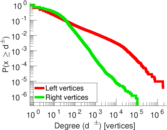### Spectral distribution of the adjacency matrix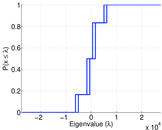### Spectral distribution of the normalized adjacency matrix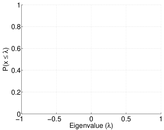### Spectral distribution of the Laplacian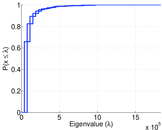### Spectral graph drawing based on the adjacency matrix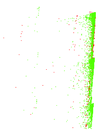### Spectral graph drawing based on the Laplacian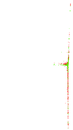### Spectral graph drawing based on the normalized adjacency matrix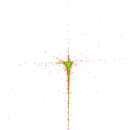### Degree assortativity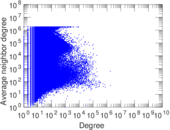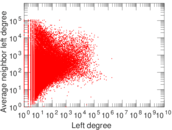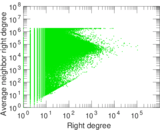### Zipf plot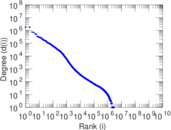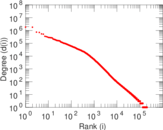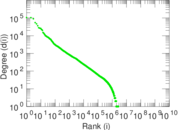### Hop distribution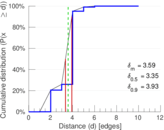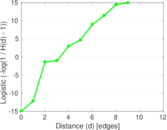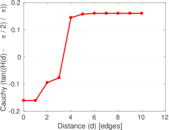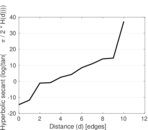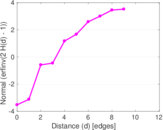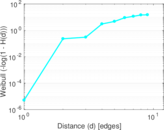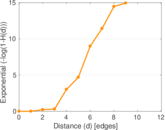### Edge weight/multiplicity distribution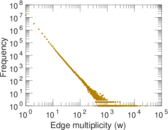### Temporal distribution